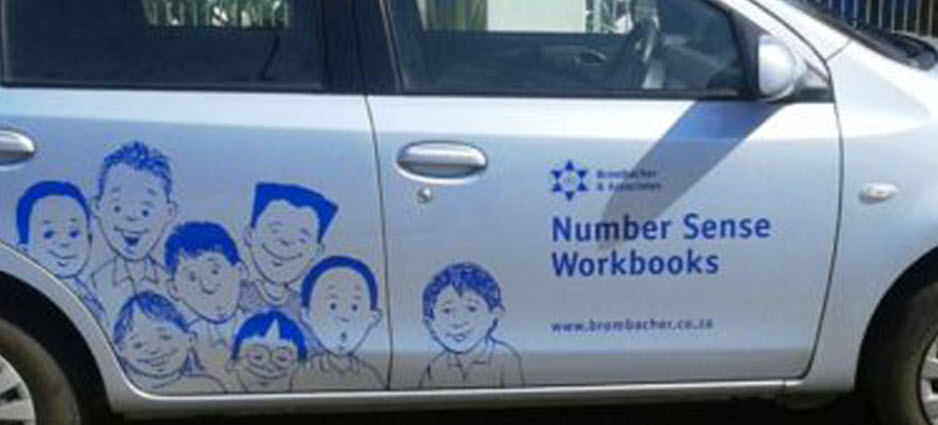5 September 2016

# Play the ‘Lucky Value’ game!• Play the 'Lucky Value' game!

Play the 'Lucky Value' game and participate in the challenges!

The “lucky value” of the number on a number plate or in a telephone number is the total of all the palindrome points. Each palindrome in the number has a point value and the “lucky value” of the number is the total of all the points. The point values of the embedded palindromes are determined as follows.

Any embedded two-digit palindrome has a point value of 1:

• The number 124456 has one embedded two-digit palindrome (44) and has a “lucky value” of 1.
• The number 122344 has two embedded two-digit palindromes (22 & 44) and has a “lucky value” of 1 + 1 = 2.

Any palindrome that consists of more than two digits has a point value equal to the number of digits in the palindrome:

• The number 123245 has one embedded three-digit palindrome (232) and has a “lucky value” of 3.
• The number 232455 has one embedded three-digit palindrome (232) and one embedded two-digit palindrome (55) and has a “lucky value” of 3 + 1 = 4.

Digits may be used for points in more than two embedded palindromes:

• The number 1223245 has one embedded one-digit palindrome (22) and one embedded three-digit palindrome (232) and has a “lucky value” of 1 + 3 = 4.

NOTE: All of the digits on the number plate or in the telephone number are considered and any other characters between the digits (e.g. spaces, dashes etc.) are all ignored

123 456 and 123-456-7890 are treated as: 123456 and 12346567890 respectively.

“Lucky value” challenges

Challenge 1: submit photographs of number plates and telephone numbers you see together with their “lucky value” and stand a chance to win a prize!

Challenge 2: submit a sequence of photographs of number plates and telephone numbers with consecutive “lucky values”, for example a series of photographs that consists of photograph of a number with a “lucky value” of 1; followed by a photograph of a number with a “lucky value” of 2; followed by a photograph of a number with a “lucky value” of 3 and so on; and stand a chance to win a prize!

To enter submit your photographs to: info@numbersense.co.za